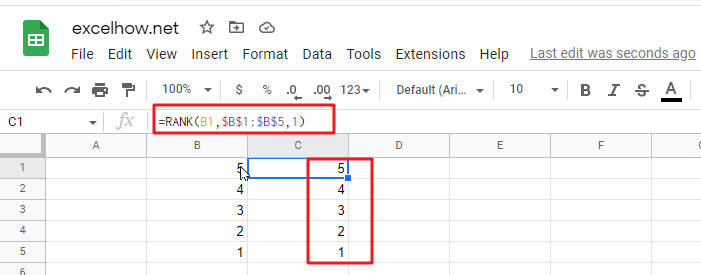# Reverse Rank Order in google sheets

This article will talk about how to rank the given data in reverse order in google sheets. You can use Google sheets RANK function to rank the data. By default, the largest value in the data table will be ranked as 1. So how do we invert this ranking order? How do we make the smallest value ranked as 1? So how do we achieve this requirement?

## Reverse Rank Order

Suppose you have a list of data and you want to sort these data and later reverse the sort order on them? If you do the ranking by manually, it is definitely not a good way to handle it. The best way is to use the RANK function to sort the data. To reverse the sort order, you just need to add a new parameter to the RANK function. The formula is as follows.

`=RANK(B1,\$B\$1:\$B\$5,1)`

As you can see from the above example, the sorting result has been reversed.### Related Functions

• Google Sheets RANK Function
The Google Sheets Rank function is used to return the ranking of a given number from a list. If there are duplicate numbers in the list, then the numbers will be in the same rank.The syntax of the Google Sheets Rank function is explained as follows:The syntax of the Google Sheets Rank function is explained as follows:= RANK (number,reference ,[order])…

Related Posts

Conditional Formatting based on Another Cell in Google Sheets/Excel

In Microsoft Excel Spreadsheet or google sheets, when you want to format a specified cell or cell range based on the value of a different cell, for example, formatting the first row based on the value of a cell in ...

Compare Two Strings in Excel/Google Sheets

This article will talk about how to compare two given strings in a Microsoft Excel spreadsheet or Google Sheets. How to compare two strings in Excel by using VBA macros to see if they are the same, if they are ...

Compare effect of (non-annual) compounding periods on growth

This article will talk about how to calculate the trend or effect of future value changes for different compounding periods in a Microsoft Excel spreadsheet or Google Sheets via a formula. What is the period of compound interest? The compounding ...

Coefficient Of Variation in Excel/Google Sheet

This article will talk about how to calculate the coefficient of variation by formula in a Microsoft Excel spreadsheet or Google Sheets.  What is the Coefficient of Variation? The coefficient of variation is the ratio of the standard deviation of ...

Phone Number Format in Excel

Sometimes when we import phone numbers from external sources, the formatting of the phone numbers is removed. Even if in some situations the phone numbers are not imported from external resource, sometimes the phone numbers in worksheet are not displayed ...

Sidebar